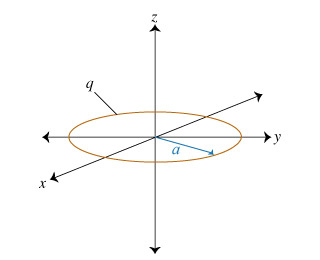# Problem: Consider a uniformly charged ring in the xy plane, centered at the origin. The ring has radius a and positive charge q distributed evenly along its circumference. Part A. What is the direction of the electric fieldat any point on the z axis?A. parallel to the x axisB. parallel to the y axisC. parallel to the z axisD. in a circle parallel to the xy plane

###### FREE Expert Solution

Consider a point with the point z, located on the positive z-axis. The ring has a radius a. An electric field vector originating from an element of charge on the ring makes an angle θ with the z-axis.

The electric field vector can be decomposed into two components. One component points in the positive z-axis. The other component points parallel to the radius.

82% (161 ratings)###### Problem Details

Consider a uniformly charged ring in the xy plane, centered at the origin. The ring has radius a and positive charge q distributed evenly along its circumference.Part A. What is the direction of the electric fieldat any point on the z axis?

A. parallel to the x axis

B. parallel to the y axis

C. parallel to the z axis

D. in a circle parallel to the xy plane